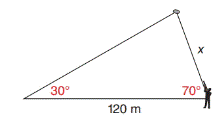Chapter 11.4, Problem 34EElementary Geometry For College St...

7th Edition
Alexander + 2 others
ISBN: 9781337614085

Solutions

Chapter
SectionElementary Geometry For College St...

7th Edition
Alexander + 2 others
ISBN: 9781337614085
Textbook Problem

In Exercises 29 to 34, use the Law of Sines or the Law of Cosines to solve each problem. Angle measures should be found to the nearest degree and areas and distances to the nearest tenth of a unit.Clay pigeons are released at an angle of 30 ∘ with the horizontal. Massimo hits one of the clay pigeons when shooting through an angle of elevation of 70 ∘ . If the point of release is 120 m from Massimo, how far ( x ) is he from the target when it is hit?To determine

To find:

The distance from the Massimo to the target.

Explanation

Formula:

The Law of Sines,

sinαa=sinβb=sinγc

Where α, β, and γ is the acute angle of the triangle, and a, b, and c is the sides of the triangle.

Calculation:

Given,

Clay pigeons are released at an angle of 30 with the horizontal. Massimo hits one of the clay pigeons when shooting through an angle of elevation of 70.

Let C be the Clay pigeons position and B be the Massimo’s position,

Clay pigeons C are released at an angle of 30 with the horizontal AB

Then A=30 and B=70

Here, AB¯=c=120 m, BC¯=a=x,

A=30andB =70

(i.e.) b=120 m., a=x, α=30 and β=70

Find x:

For any triangle,

α+β+γ=180

30+70+γ=180

100+γ=180<

Still sussing out bartleby?

Check out a sample textbook solution.

See a sample solution

The Solution to Your Study Problems

Bartleby provides explanations to thousands of textbook problems written by our experts, many with advanced degrees!

Get Started

Identify the four steps of a hypothesis test as presented in this chapter.

Statistics for The Behavioral Sciences (MindTap Course List)

Given that the point P(2, 3) lies on the line 2x + ky + 10 = 0, find k.

Applied Calculus for the Managerial, Life, and Social Sciences: A Brief Approach

Multiply the following numbers and verify your answers. 4.

Contemporary Mathematics for Business & Consumers

The graph of is: a) b) c) d)

Study Guide for Stewart's Multivariable Calculus, 8th

In Exercises 116, determine whether the argument is valid. pq~pq

Finite Mathematics for the Managerial, Life, and Social Sciences